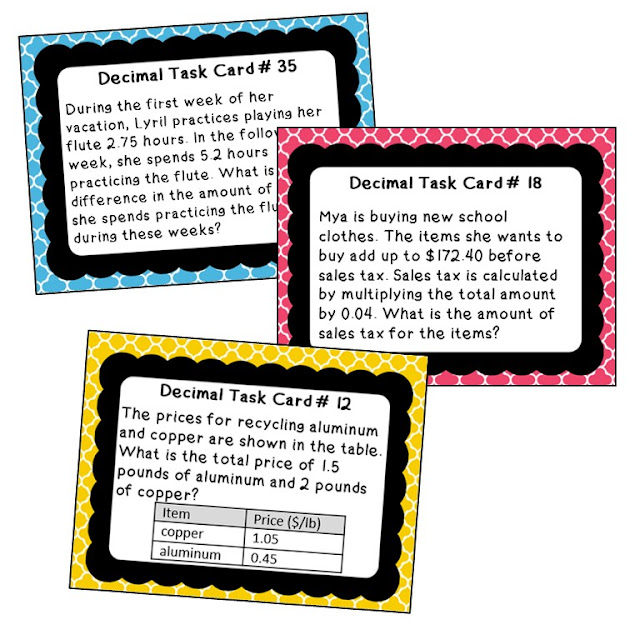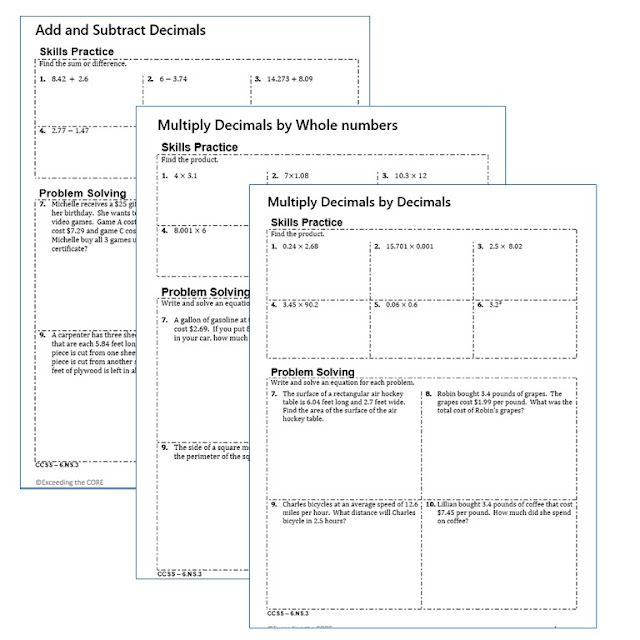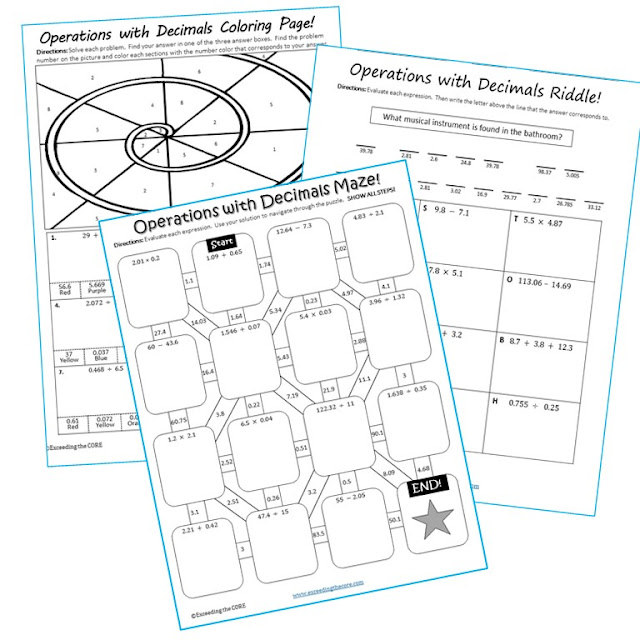## DECIMALS Math BUNDLE - Task Cards, Error Analysis, Word Problem Solving Graphic Organizer, Homework Practice Worksheets

This Unit Resource BUNDLE includes (a total of 150 decimal skills practice and real-world word problems).
10 error analysis activities
10 problem solving graphic organizers
5 homework practice worksheets,
1 maze activity
1 riddle activity
1 coloring page,
4 guided note pages

The resources in this bundle are perfect for warm-ups, cooperative learning, spiral review, math centers, end of year review, assessment prep and homework.

Topics included:
✔ Multiply Decimals by Whole Numbers
✔ Multiply Decimals by Decimals
✔ Divide Decimals by Decimals

✔ Divide Decimals by Whole Numbers

Each page has a real-world word problems that is solved incorrectly. Students have to identify the error, provide the correct solution and share a helpful strategy for solving the problem.Operations with Decimals Error Analysis Activity

Each task card has a real-world word problem. Students can work on these cards individually, with partners or in groups.Operations with Decimals Task Cards

Each worksheet presents students with a real-word problem. Students must then organize the information using a problem-solving graphic organizer. Students are prompted to identify the important information in the word problem, solve, justify their work and explain their solution.Multi-Digit Decimal Operations Word Problems (Graphic Organizers)

Each worksheet includes two sections, Skills Practice and Problems Solving. The SKILLS PRACTICE section of each worksheet includes 6 computational problems to help students sharpen their skills. The WORD PROBLEM section of each worksheet allows students to apply their understanding of the topic to solve 4 real-world problems.Add, Subtract, Multiply Divide Decimals Worksheets

A maze, riddle and coloring page is also included. All 3 of these activities allow students to practice this skill while incorporating fun into the classroom!Operations with Decimals Maze Riddle and Coloring Page

These Guided Notes include essential vocabulary, key concepts and practice questions. Students answer the questions, and then color, doodle or embellish these notes. These guided notes are perfect for interactive notebooks or mini-anchor charts.Add, Subtract, Multiply Divide Decimal Guided Notes